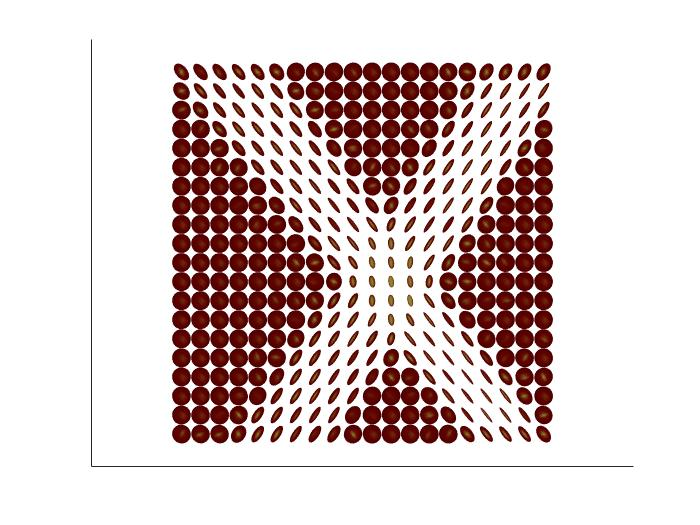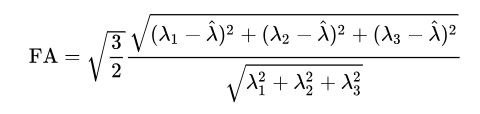Warning

## Lab 11 : Diffusion weighted MRI

Today we look at Diffusion-weighted Imaging - one specific MR modality. With dMRI, we can use gradient direction to measure the movement of molecules in that direction. If there is no obstacle at the measuring point, then the signals at different gradients will not differ. This is not the case when we have obstacles in our way, such as axons of nerve connections. The diffusion takes place along the unrestricted direction and gets restricted in transverse directions. Thus, a signal from a sufficient number of diffusion gradients can tell us about the structure and orientation of the tissue at a given point.

One of the basic models is Diffusion Tensor Imaging (DTI), uses symmetric tensor (3 × 3 matrix) to determine the main direction of diffusion. The diffusion of water molecules is modeled as a three-dimensional Gaussian process.

#### Assignment

1. From File diffusion_data.mat load 4D image (dimension X,Y,Z=1,grad)dwimage. The information about gradients strength is stored in bvals and that of directions in bvecs array. This data contains a 2D tensor image, as shown below. The individual tensors are in 3D indicating water diffusion in three directions at each position.

Find the coefficients of the symmetric tensor in Stejskal-Tanner equation which can be obtained using least square fitting method. Force the negative eigenvalues to small positive number (eps) to ensure positive eigenvalues. Use the script plotdti.m with arguments plotDTI(T(3,3,x,y), size) to the display tensor image. Adjust the second argument “size” of the order of $10^{-2}$ to decrease/increase the size of tensors. What does it tells about the four types of regions of tissues on the basis of anisotropy and orientation? Is this Gaussian model sufficient for intersection region?2. From the tensors, determine the values ​​of fractional anisotropy (FA), mean diffusivity (MD) and radial diffusivity (RD) maps for the given tensor image. Given real image realbrainimage.zip(.nii format) with b-values and b-vecs. Use niftiread() Matlab function to read the file and SPM or MITK to select the slice where Corpus Callosum (CC: the longest white matter structure) is visible. Further, use imrect() Matlab function to select the rectangular coordinates of the section of CC (white matter), gray matter, and corticospinal fluid region (CSF). Display FA and MD maps of the slice of image and compute the mean and standard deviation of FA values in these three rectangular selected regions? 

3. For synthetic images (in question 1), Create a diffusion data visualization of the main diffusion direction in each voxel (the eigenvector belonging to the largest eigenvalue) and draw it (easiest to do this using the quiver function ), do not consider background region where FA will be near 0. Are there places in the image where the main direction information calculated using DTI is insufficient in providing actual directions of fibers? [1.5]

### Instructions

#### (A) Reconstruction of diffusion tensor image

Further, the given data- dwimage, contains for each gradient one 2D section of size 20 × 20, the gradient scheme contains 3x unweighted image ( $b_0$) and 30x gradient with power b = 1000 . In total, it has dimensions of 20 x 20 x 1 x 33. Notice N=33. The corresponding gradient directions are stored as 33 × 3 matrices bvecs. We can draw directions with

scatter3(bvecs(1,:), bvecs(2,:), bvecs(3,:), 50, 'filled');

The following Stejskal Tanner equation describes the diffusion at each position:
$S_k=S_{0}\exp(-b(g_{k})^t T (g_{k})))$
where, $k= 1..N$, $g_{k}=(g_{(k,x)}, g_{(k,y)},g_{(k,z)})^t$
T is the diffusion tensor and b is a constant depicting the the magnitude of the sensitizing gradients.
In log-space this equation is a linear equation which can be solved using least square method as done in home work 1.
The value of $S_{0}$= depends upon the data range if it's equal to [0,1] then set it =1 and if [0, 255] then set it =255; $\log(S_{0})-\log(S_{k})=b(g_{k})^t T (g_{k})$
which can be written as-
$AX=Y$ where,
$A_{N\times6}(;,i)=[g_{(i,x)}^2 , 2g_{(i,x)}g_{(i,y)}, 2g_{(i,x)}g_{(i,z)} ,...,g_{(i,z)}^2 ]$(knowns: monomials of gradient(1×3) vector)
$X_{6\times1}=b[T_{xx},T_{xy},T_{xz},T_{yy},T_{yz},T_{zz}]^t$ (Unknown coefficients to find ?)
$Y_{N\times1}=\log(S_0)-\log(S_k)$(Known attenuation of signal)

Use eig Matlab function to find 3 eigen values and eigen vectors. The Tensor(x,y) is 3×3 matrix at each (x,y)location obtained from above equation.

[eigVec, eigVal]=eig(Tensor(x,y))

#### (B) Eigen analysis and scalar anisotropies

quiver(X,Y,U,V)
axis equal
The vectors X and Y represent the location of the base of each arrow, and U and V represent the directional components of each arrow. The principal direction is the eigenvector corresponding to the largest eigenvalue. On a uniform grid of (x,y) coordinates, calculate the principal directions $(u,v)$ and display them using the 'quiver' command. You may follow this link to know the formulas for various anisotropies for h/w tasks and in general about DTI :dwMR1 anddwMR2
We want to further reduce the multi-dimensional information of a tensor to a set of representative scalar values. One widely used measure is the fractional anisotropy(FA) describes how much a single direction prevails among others (in other words: how big is the discrepancy between the largest and the two other eigenvalues)The mean diffusivity (MD) shows the magnitude of diffusion within tissue and is defined as the mean value of the three eigenvalues $$MD = \frac{\lambda_1 + \lambda_2 + \lambda_3}{3}$$ The radial diffusivity can be interpreted as the coherence of the tissue and is defined as the mean value of the directions orthogonal to the principal diffusion direction (i.e. the largest eigenvalue). $$RD = \frac{\lambda_2 + \lambda_3}{2}$$